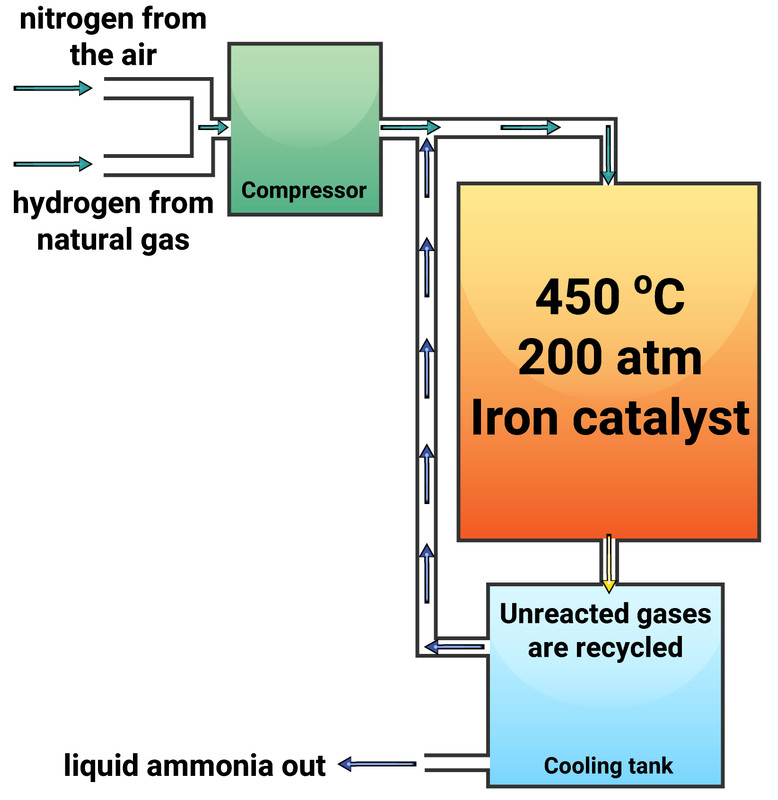# Reversible Reactions and Dynamic Equilibrium

### Reversible Reactions

You should know that in a chemical reaction, reactants react to make products:

A + B → C + D

However, in some chemical reactions the products can react together to reform the original reactants. This is called a reversible reaction, and we use a different arrow to represent it:

A + B ⇌ C + D

The top half of the arrow represents the forward reaction, where A and B makes C and D. The bottom half of the arrow represents the reverse reaction (sometimes called a backwards reaction), when C and D recombine to make A and B.

One of these reactions (forward or reverse) will release energy to the surroundings (exothermic), and the other will take in energy (endothermic).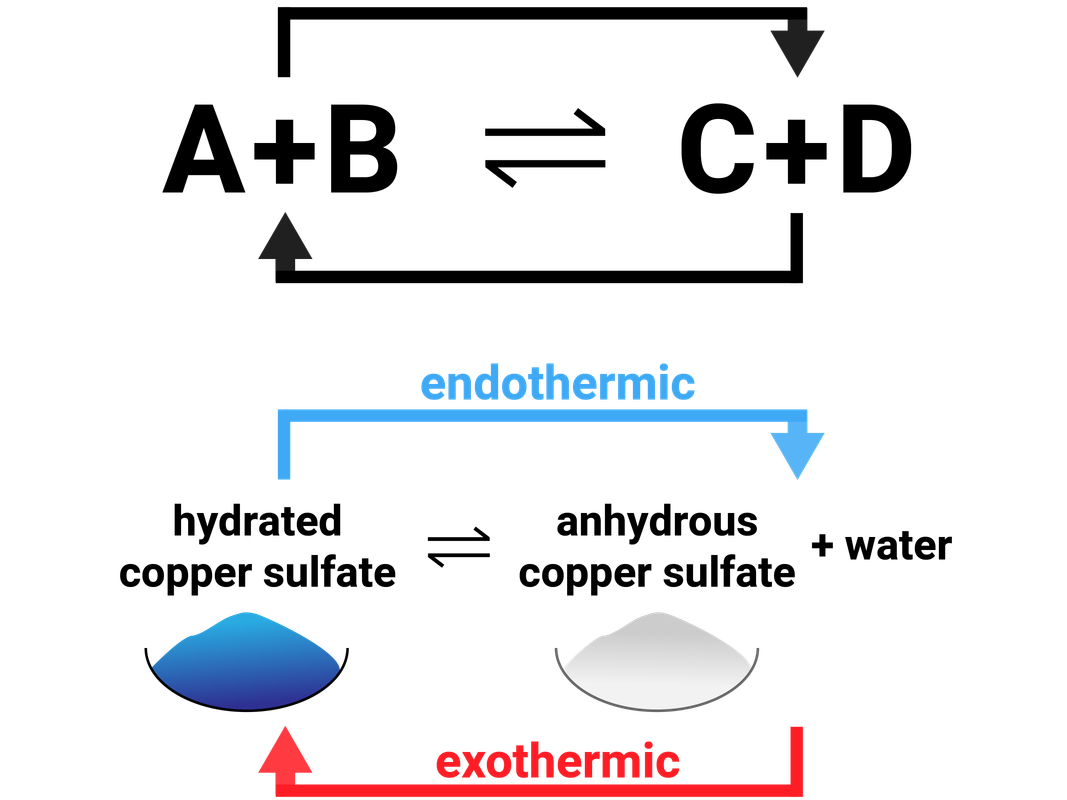### Dynamic equilibrium

Equilibrium is reached when the forward and reverse reactions, of a reversible reaction, occur at the same rate (the reaction must be in a closed system).

When the forward and reverse rates balance, and are equal, it can appear as if the reaction isn't doing anything - and is finished. What actually is happening is that the reactants are being converted into products at the same rate the products are converted back into reactants.

The concentrations of the reactants vs products are not necessarily equal, and there is usually more of one than the other:

• if the concentration of the reactants is higher than products, we say the equilibrium lies to the left
• if the concentration of the products is higher than the reactants, we say the equilibrium lies to the right

Dynamic equilibrium is when the forward and reverse reactions are still occurring (dynamic), but the substances remain in balance (equilibrium).

Higher Tier

If a system is at equilibrium and a change is made to any of the conditions (temperature, concentration, pressure), then that system will respond to counteract the change.
This is called Le Chatelier's Principle.

Changing the concentration, temperature and pressure of a reaction system can make a big change to where the equilibrium lies, and industry uses this principle regularly to increase the amount of product they make (for the best profits!).

Changing concentration

• if you add more reactant, the equilibrium will shift to the right to reduce the concentration of reactant (and make more product)
• if you remove some of the product, the equilibrium will shift to the right to increase the concentration of the product

Changing temperature

• if the temperature is increased then the equilibrium position will shift to reduce the temperature (so will favour the endothermic reaction)
• if the temperature is decreased then the equilibrium position will shift to increase the temperature (so will favour the exothermic reaction)

Changing pressure

• if you increase the pressure then the equilibrium will shift to reduce it (by favouring which ever side of the reaction has the fewest molecules of gas)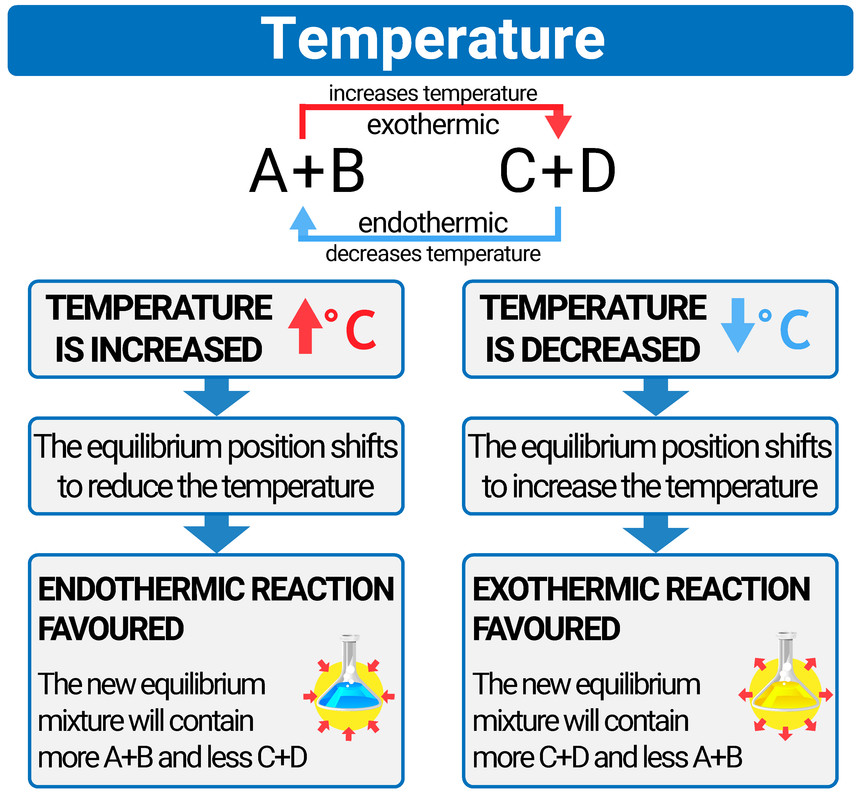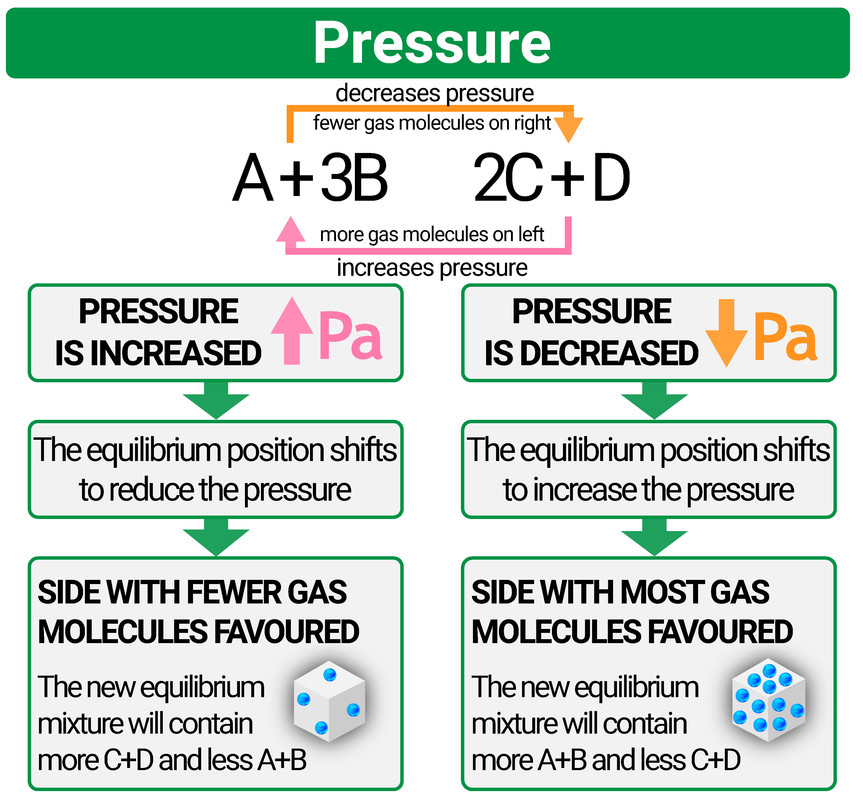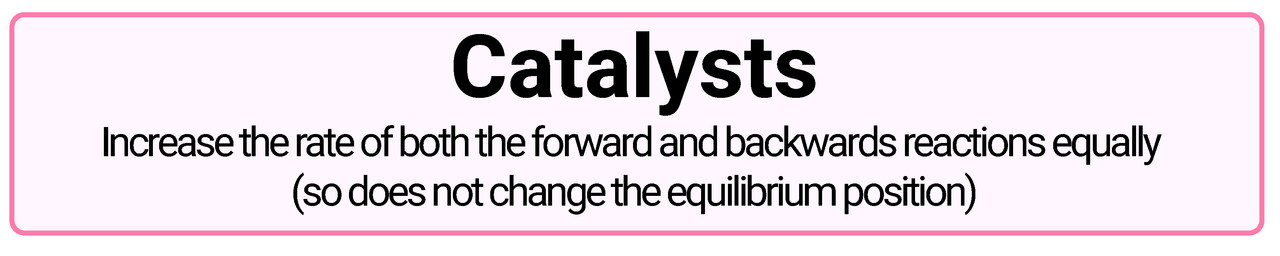### The Haber Process

nitrogen + hydrogen ⇌ ammonia

N2 + 3H2 ⇌ 2NH3

The Haber Process is used to produce ammonia, which can be used to manufacture nitrogen-based fertilisers. This process is a reversible reaction between nitrogen (extracted from the air) and hydrogen (obtained from natural gas). This reaction can reach a dynamic equilibrium.

The conditions for the Haber Process are:

• temperature of 450 °C
• pressure of 200 atmospheres (atm)
• iron catalyst

Higher Tier

A catalyst will increase the rate of both reactions equally, and so does not change the yield of the reaction. The reasons why the other above conditions are used, is given below:

Temperature of 450 °C

• forward reaction is exothermic, so a lower temperature favours the forward reaction to maximise yield
• through heating particles will have more energy, so more frequent, successful collisions between particles
• a compromise is made on the temperature

Pressure of 200 atm

• the forward reaction turns 4 moles ('volumes') of gas into 2 moles of gas
• increasing the pressure will favour the forward reaction to maximise the yield (as this forces the particles to take up less volume)
• to maintain this high pressure, lots of energy is needed - so high costs